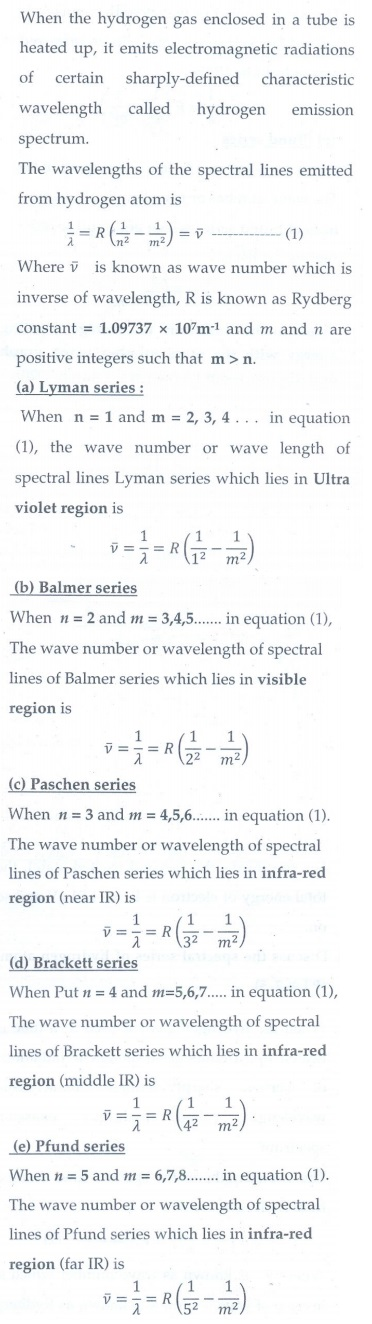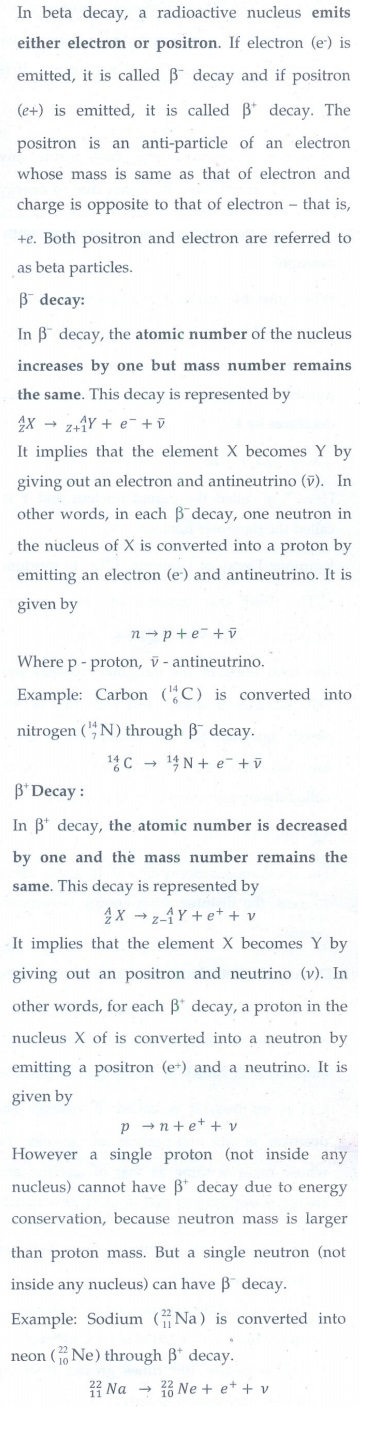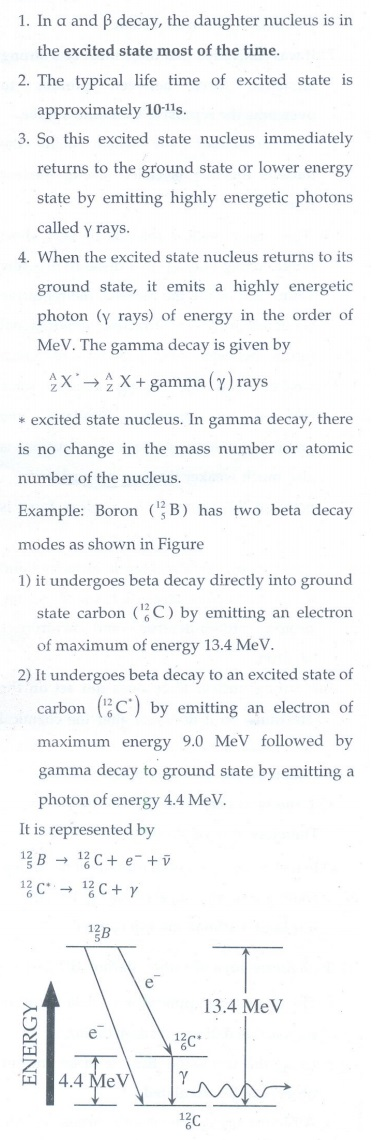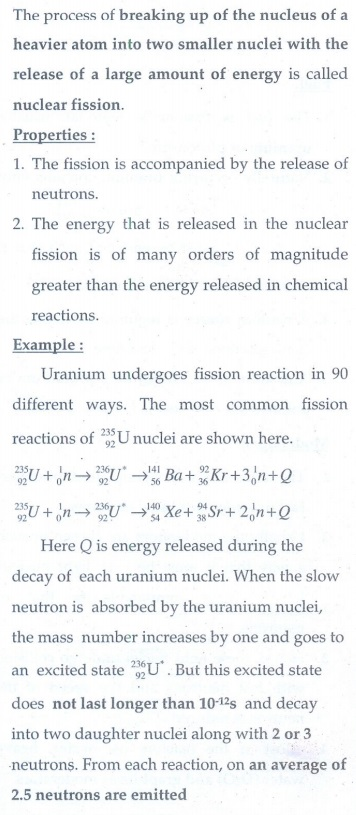Home | | Physics 12th Std | Long answer questions

## Chapter: 12th Physics : UNIT 9 : Atomic and Nuclear Physics

Physics : Atomic and Nuclear Physics: Book Back Important Questions, Answers, Solutions: Long answer questions

1. Explain the J.J. Thomson experiment to determine the specific charge of electron.2. Discuss the Millikan’s oil drop experiment to determine the charge of an electron.3. Derive the energy expression for hydrogen atom using Bohr atom model.4. Discuss the spectral series of hydrogen atom.5. Explain the variation of average binding energy with the mass number by graph and discuss its features.6. Explain in detail the nuclear force.7. Discuss the alpha decay process with example.8. Discuss the beta decay process with examples.9. Discuss the gamma decay process with example.10. Obtain the law of radioactivity.11. Discuss the properties of neutrino and its role in beta decay.12. Explain the idea of carbon dating.13. Discuss the process of nuclear fission and its properties.14. Discuss the process of nuclear fusion and how energy is generated in stars?15. Describe the working of nuclear reactor with a block diagram.16. Explain in detail the four fundamental forces.17. Briefly explain the elementary particles of nature.Tags : Atomic and Nuclear Physics | Physics , 12th Physics : UNIT 9 : Atomic and Nuclear Physics
Study Material, Lecturing Notes, Assignment, Reference, Wiki description explanation, brief detail
12th Physics : UNIT 9 : Atomic and Nuclear Physics : Long answer questions | Atomic and Nuclear Physics | Physics